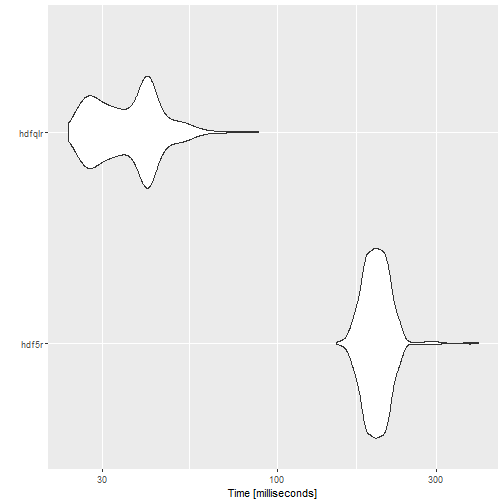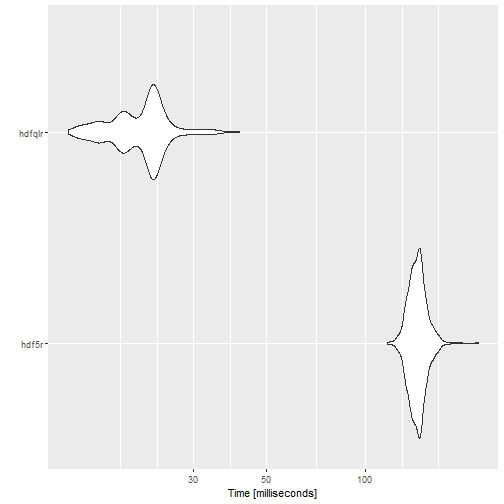# Benchmarking hdfqlr

#### 2021-06-09

This document provides some benchmarks for comparing the performance of hdfqlr to the hdf5r package. This vignette previously included comparisons to the now-deprecated h5 package. Other packages that provide read (but not write) support for HDF files were not tested.

library(hdfqlr)
library(hdf5r)
library(microbenchmark)

## Writing HDF datasets

write_hdfqlr = function() {
test.file = tempfile(fileext = ".h5")
hql_create_file(test.file)
hql_use_file(test.file)
for (i in paste0("vector", 1:6)) {
write("DATASET", runif(10000), i)
}
hql_close_file(test.file)
}

write_hdf5r = function() {
test.file = tempfile(fileext = ".h5")
# create hdf5 file (6 vectors with 10k random numbers each)
h5file = hdf5r::H5File$new(test.file, "w") for (i in paste0("vector", 1:6)) { h5file[[i]] = runif(10000) } h5file$close_all()
}

write.benchmark = microbenchmark(
"hdf5r" = write_hdf5r(),
"hdfqlr" = write_hdfqlr(),
times = 1000L,
unit = 'ms'
)
expr min lq mean median uq max neval
hdf5r 150.1851 185.27945 199.61208 198.1042 211.18990 399.0265 1000
hdfqlr 23.8104 29.05005 36.77295 37.1426 41.68425 88.0541 1000plot of chunk write-benchmark

## Reading HDF datasets

tf = tempfile(fileext = ".h5")
hql_create_file(tf)
hql_use_file(tf)
sets = paste0("vector", 1:6)
for (i in sets) {
hql_write_dataset(runif(10000), i)
}
hql_close_file(tf)

read_hdfqlr = function(file, sets) {
hql_use_file(file)
result = lapply(sets, hql_read_dataset)
hql_close_file(file)
result
}

read_hdf5r = function(file, sets) {
h5file = hdf5r::H5File$new(file, "r") result = lapply(sets, function(i) h5file[[i]][]) h5file$close_all()
result
}

)